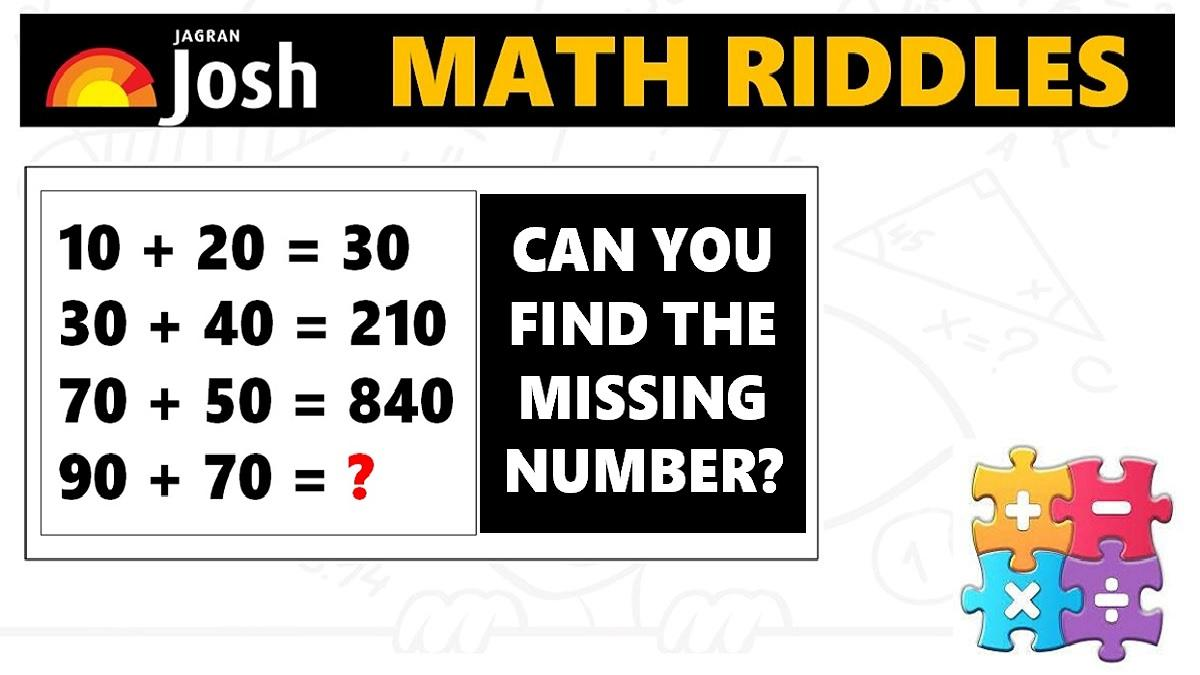# Math Riddles: Can You Find The Next Number In These Math Puzzle Series?

Math Riddles Challenge: You are 100% genius if you can find the missing numbers in these mathematics puzzle in less than 1 minute each?Math Riddles: Can You Find The Next Number In These Math Puzzle Series?

Math Riddles: Only 1% of geniuses can solve these mathematics puzzles. Each of the puzzles is a set of equations where we have to find the missing number in the place of the question mark. These are some tricky logical math puzzles that will require logical reasoning. The challenge is to solve them each in 1 minute. If you are looking to boost your logical reasoning and quantitative aptitude preparation, check out these puzzle series.

## Solution

### Maths Logic Puzzle #1

Explanation:

The logic in this mathematics puzzle: Formula -> x + y * 1st Number Tenth Place

=> 10 + 20 = 30, How?

We will start solving each row of equation:

Row 1 -> 10 + 20 = 30 => x + y * 1st Number Tenth Place => 10 + 20 * 1 = 30 * 1 = 30

Row 2 -> 30 + 40 = 210 => x + y * 1st Number Tenth Place => 30 + 40 * 3 = 70 * 3 = 210

Row 3 -> 70 + 50 = 840 => x + y * 1st Number Tenth Place => 70 + 50 * 7 = 120 * 7 = 840

Similarly, we shall solve the last row to find the missing number.

Row 4 -> 90 + 70 = ? => x + y * 1st Number Tenth Place => 90 + 70 * 9 = 160 * 9 = 1440

Explanation:

## Tell us in comments: Did you solve these mathematics puzzles in less than 1 minute each?

Check out more math puzzles!

Also Read: Math Riddles: Can You Find The Next Number In These Math Puzzle Series?

Also Read: Math Riddles: Only 1 out 5 People Can Solve These 5-Minute Maths Puzzles

Also Read: Math Riddles: 5-Minute Maths Puzzles, Find the Answers in Less than 1 Minute

Get the latest General Knowledge and Current Affairs from all over India and world for all competitive exams.
खेलें हर किस्म के रोमांच से भरपूर गेम्स सिर्फ़ जागरण प्ले पर